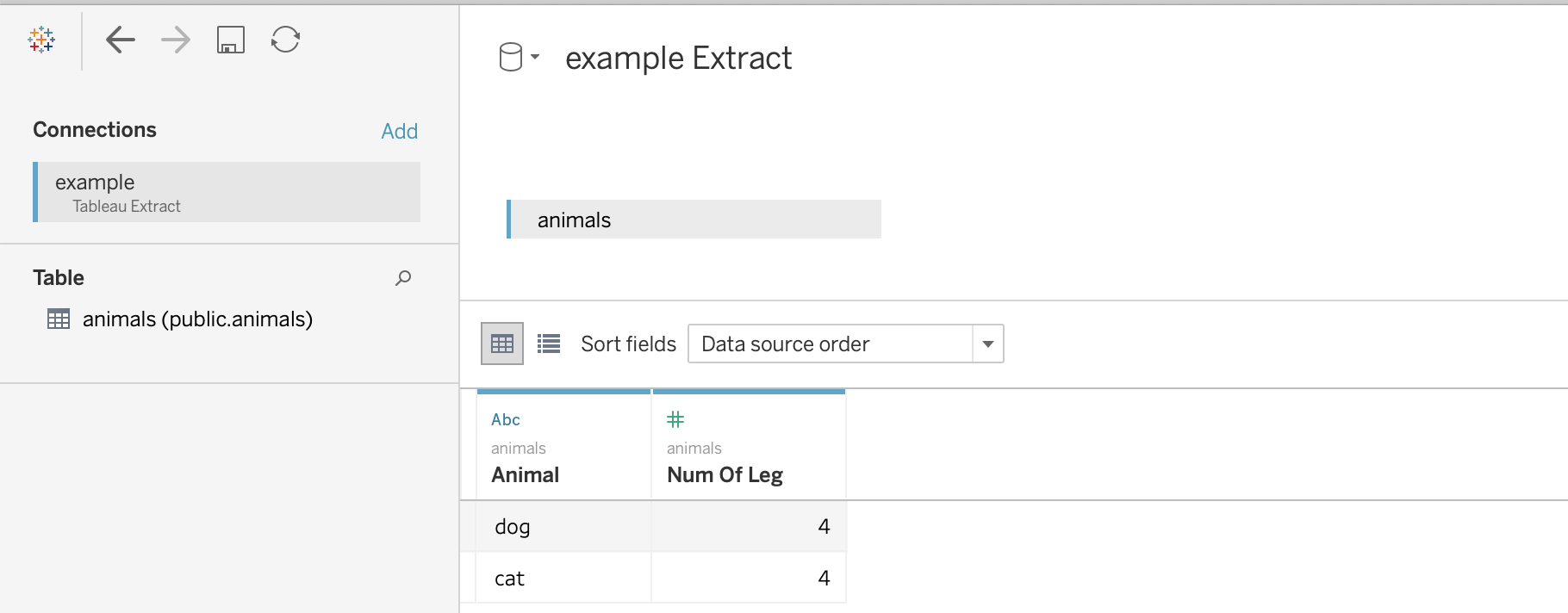# Usage Examples¶

## Writing to a Hyper Extract¶

```import pandas as pd
import pantab

df = pd.DataFrame([
["dog", 4],
["cat", 4],
], columns=["animal", "num_of_legs"])

pantab.frame_to_hyper(df, "example.hyper", table="animals")
```

The above example will write out to a file named “example.hyper”, which Tableau can then report off of.```import pantab

df = pantab.frame_from_hyper("example.hyper", table="animals")
print(df)
```

## Working with Schemas¶

By default tables will be written to the “public” schema. You can control this behavior however by specifying a `tableauhyperapi.TableName` when reading / writing extracts.

```import pandas as pd
import pantab
from tableauhyperapi import TableName

# Let's write somewhere besides the default public schema
table = TableName("not_the_public_schema", "a_table")

df = pd.DataFrame([
["dog", 4],
["cat", 4],
], columns=["animal", "num_of_legs"])

pantab.frame_to_hyper(df, "example.hyper", table=table)

# Can also be round-tripped
df2 = pantab.frame_from_hyper("example.hyper", table=table)
```

Note

If you want to publish a hyper file using the Tableau Server REST API and you’re using using a version prior to 2020.1 you’ll need to have a single table named `Extract` that uses the `Extract` schema (`Extract.Extract`).

## Reading and Writing Multiple Tables¶

`frames_to_hyper` and `frames_from_hyper` can write and return a dictionary of DataFrames for Hyper extract, respectively.

```import pandas as pd
import pantab
from tableauhyperapi import TableName

dict_of_frames = {
"table1": pd.DataFrame([[1, 2]], columns=list("ab")),
TableName("non_public_schema", "table2"): pd.DataFrame([[3, 4]], columns=list("cd")),
}

pantab.frames_to_hyper(dict_of_frames, "example.hyper")

# Can also be round-tripped
result = pantab.frames_from_hyper("example.hyper")
```

Note

While you can write using `str`, `tableauhyperapi.Name` or `tableauhyperapi.TableName` instances, the keys of the dict returned by `frames_from_hyper` will always be `tableauhyperapi.TableName` instances

## Appending Data to Existing Tables¶

By default, `frame_to_hyper` and `frames_to_hyper` will fully drop and reloaded targeted tables. However, you can also append records to existing tables by supplying `table_mode="a"` as a keyword argument.

```import pandas as pd
import pantab

df = pd.DataFrame([
["dog", 4],
["cat", 4],
], columns=["animal", "num_of_legs"])

pantab.frame_to_hyper(df, "example.hyper", table="animals")

new_data = pd.DataFrame([["moose", 4]], columns=["animal", "num_of_legs"])

# Instead of overwriting the animals table, we can append via table_mode
pantab.frame_to_hyper(df, "example.hyper", table="animals", table_mode="a")
```

Please note that `table_mode="a"` will create the table(s) if they do not already exist.

## Issuing SQL queries¶

New in version 2.0.

With `frame_from_hyper_query`, one can execute SQL queries against a Hyper file and retrieve the resulting data as a DataFrame. This can be used, e.g. to retrieve only a part of the data (using a `WHERE` clause) or to offload computations to Hyper.

```import pandas as pd
import pantab

df = pd.DataFrame([
["dog", 4],
["cat", 4],
["moose", 4],
["centipede", 100],
], columns=["animal", "num_of_legs"])

pantab.frame_to_hyper(df, "example.hyper", table="animals")

# Read a subset of the data from the Hyper file
query = """
SELECT animal
FROM animals
WHERE num_of_legs > 4
"""
df = pantab.frame_from_hyper_query("example.hyper", query)
print(df)

# Let Hyper do an aggregation for us - it could also do joins, window queries, ...
query = """
SELECT num_of_legs, COUNT(*)
FROM animals
GROUP BY num_of_legs
"""
df = pantab.frame_from_hyper_query("example.hyper", query)
print(df)
```

New in version 2.0.

For convenience, pantab’s functions internally spawn a HyperProcess. In case you prefer to spawn your own `HyperProcess`, you can supply it to pantab through the `hyper_process` keyword argument.

By using your own `HyperProcess`, you have full control over all its startup paramters. In the following example we use that flexibility to:

• enable telemetry, thereby making sure the Hyper team at Tableau knows about our use case and potential issues we might be facing
• disable log files, as we operate in some environment with really tight disk space
• opt-in to the new Hyper file format

By reusing the same `HyperProcess` for multiple operations, we also save a few milliseconds. While not noteworthy in this simple example, this might be a good optimization in case you call `frame_to_hyper` repeatedly in a loop.

```import pandas as pd
import pantab
from tableauhyperapi import HyperProcess, Telemetry

df = pd.DataFrame([
["dog", 4],
["cat", 4],
], columns=["animal", "num_of_legs"])

parameters = {"log_config": "", "default_database_version": "1"}
with HyperProcess(Telemetry.SEND_USAGE_DATA_TO_TABLEAU, parameters=parameters) as hyper:
# Insert some initial data
pantab.frame_to_hyper(df, "example.hyper", table="animals", hyper_process=hyper)

# Append additional data to the same table using `table_mode="a"`
new_data = pd.DataFrame([["moose", 4]], columns=["animal", "num_of_legs"])
pantab.frame_to_hyper(df, "example.hyper", table="animals", table_mode="a", hyper_process=hyper)
```

## Providing your own Hyper Connection¶

New in version 2.0.

In order to interface with Hyper, pantab functions need a HyperAPI Connection to interface with Hyper. For convenience, pantab creates those connections implicitly for you. However, establishing a connection is not for free, and by reusing the same `Connection` for multiple operations, we can save time. Hence, pantab also allows you to pass in a HyperAPI connection instead of the name / location of your Hyper file.

```import pandas as pd
import pantab
from tableauhyperapi import HyperProcess, Telemetry, Connection, CreateMode

df = pd.DataFrame([
["dog", 4],
["cat", 4],
["centipede", 100],
], columns=["animal", "num_of_legs"])
path = "example.hyper"

with HyperProcess(Telemetry.DO_NOT_SEND_USAGE_DATA_TO_TABLEAU) as hyper:
pantab.frames_to_hyper({"animals": df}, path, hyper_process=hyper)

with Connection(hyper.endpoint, path, CreateMode.NONE) as connection:
query = """
SELECT animal
FROM animals
WHERE num_of_legs > 4
"""
many_legs_df = pantab.frame_from_hyper_query(connection, query)
print(many_legs_df)

all_animals = pantab.frame_from_hyper_query(connection, table="animals")
print(all_animals)
```